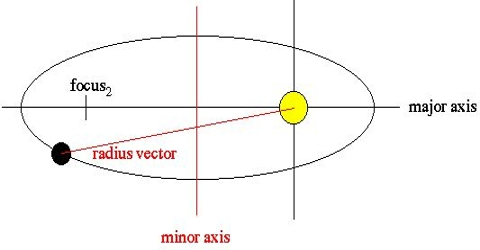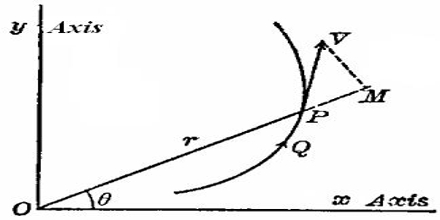# Radius Vector: Definition and Explanation

Radius vector a straight line amalgamation the source of a vector, positioned at the intersection of two coordinates, to a given point lying in the same plane. The radius vector (ř) is the vector from some origin to the position of the particle at some time (t). Typically, ř(t) is the trajectory, so to speak of the particle, so the derivative with respect to time is the velocity:

vt = dř/dt. [where v is the magnitude of the velocity (speed)]

Because the position is constantly changing, the velocity as well as the angle changes with respect to the origin. The radius extends from this origin to the position of the object.In many cases, the position of something is expressed by position vector. It is called radius vector ř.

So, if the positions coordinate of a point P is, (x, y, z), then radius vector is expressed as:

ř = x î + y ĵ + z ƙ;

and its modulus will be; r = |ř| = √(x2 + y2 + z2)